Anúncio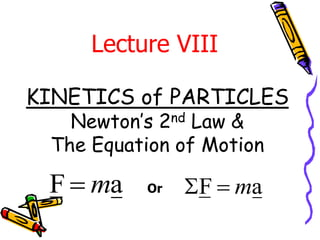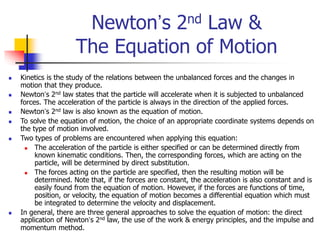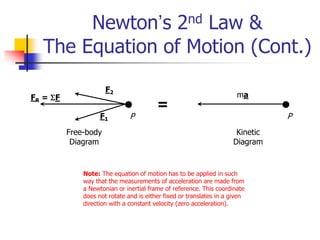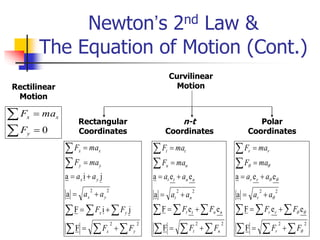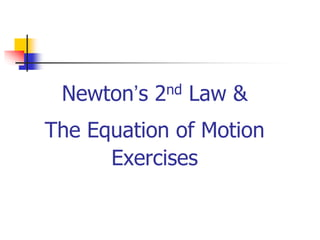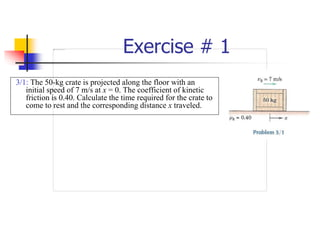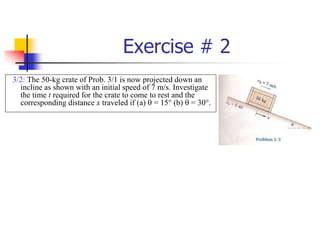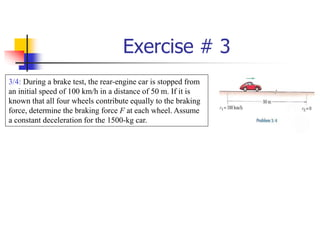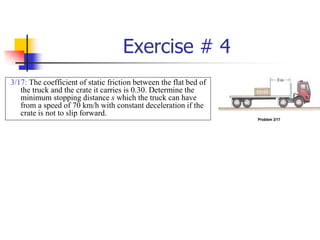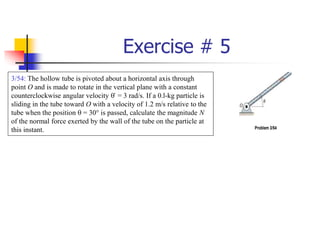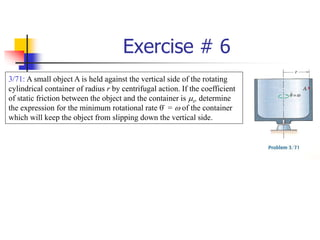1 de 11
Anúncio

### 4933896.ppt

1. KINETICS of PARTICLES Newton’s 2nd Law & The Equation of Motion Lecture VIII a F m  a F m   Or
2. Newton’s 2nd Law & The Equation of Motion  Kinetics is the study of the relations between the unbalanced forces and the changes in motion that they produce.  Newton’s 2nd law states that the particle will accelerate when it is subjected to unbalanced forces. The acceleration of the particle is always in the direction of the applied forces.  Newton’s 2nd law is also known as the equation of motion.  To solve the equation of motion, the choice of an appropriate coordinate systems depends on the type of motion involved.  Two types of problems are encountered when applying this equation:  The acceleration of the particle is either specified or can be determined directly from known kinematic conditions. Then, the corresponding forces, which are acting on the particle, will be determined by direct substitution.  The forces acting on the particle are specified, then the resulting motion will be determined. Note that, if the forces are constant, the acceleration is also constant and is easily found from the equation of motion. However, if the forces are functions of time, position, or velocity, the equation of motion becomes a differential equation which must be integrated to determine the velocity and displacement.  In general, there are three general approaches to solve the equation of motion: the direct application of Newton’s 2nd law, the use of the work & energy principles, and the impulse and momentum method.
3. Newton’s 2nd Law & The Equation of Motion (Cont.) Kinetic Diagram Free-body Diagram = ma F2 F1 FR = F P P Note: The equation of motion has to be applied in such way that the measurements of acceleration are made from a Newtonian or inertial frame of reference. This coordinate does not rotate and is either fixed or translates in a given direction with a constant velocity (zero acceleration).
4. Newton’s 2nd Law & The Equation of Motion (Cont.) 0     y x x F ma F 2 2 2 2 F j i F a j i a                   y x y x y x y x y y x x F F F F a a a a ma F ma F Rectilinear Motion Curvilinear Motion Rectangular Coordinates n-t Coordinates Polar Coordinates 2 2 2 2 F e e F a e e a                   n t n n t t n t n n t t n n t t F F F F a a a a ma F ma F 2 2 2 2 F e e F a e e a                           F F F F a a a a ma F ma F r r r r r r r r
5. Newton’s 2nd Law & The Equation of Motion Exercises
6. Exercise # 1 3/1: The 50-kg crate is projected along the floor with an initial speed of 7 m/s at x = 0. The coefficient of kinetic friction is 0.40. Calculate the time required for the crate to come to rest and the corresponding distance x traveled.
7. Exercise # 2 3/2: The 50-kg crate of Prob. 3/1 is now projected down an incline as shown with an initial speed of 7 m/s. Investigate the time t required for the crate to come to rest and the corresponding distance x traveled if (a)  = 15° (b)  = 30°.
8. Exercise # 3 3/4: During a brake test, the rear-engine car is stopped from an initial speed of 100 km/h in a distance of 50 m. If it is known that all four wheels contribute equally to the braking force, determine the braking force F at each wheel. Assume a constant deceleration for the 1500-kg car.
9. Exercise # 4 3/17: The coefficient of static friction between the flat bed of the truck and the crate it carries is 0.30. Determine the minimum stopping distance s which the truck can have from a speed of 70 km/h with constant deceleration if the crate is not to slip forward. Problem 3/17
10. Exercise # 5 3/54: The hollow tube is pivoted about a horizontal axis through point O and is made to rotate in the vertical plane with a constant counterclockwise angular velocity  . = 3 rad/s. If a 0.l-kg particle is sliding in the tube toward O with a velocity of 1.2 m/s relative to the tube when the position  = 30° is passed, calculate the magnitude N of the normal force exerted by the wall of the tube on the particle at this instant. Problem 3/54
11. Exercise # 6 3/71: A small object A is held against the vertical side of the rotating cylindrical container of radius r by centrifugal action. If the coefficient of static friction between the object and the container is ms, determine the expression for the minimum rotational rate  . = w of the container which will keep the object from slipping down the vertical side.
Anúncio